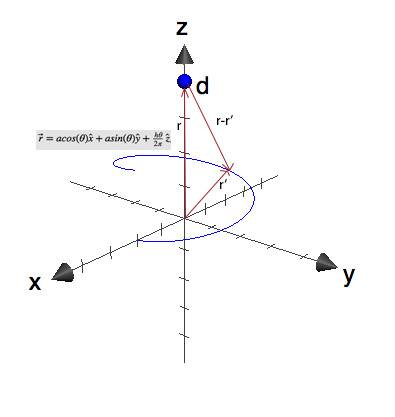# Electric Field Above Helix

tylerscott

## Homework StatementA charge Q is uniformly distributed with linear density λ over a helix parameterized as $\vec{r}=acos(\theta)\hat{x}+asin(\theta)\hat{y}+ \frac{ h\theta}{2 \pi}\hat{z}$, where a and h are positive constants, and 0<∏<2∏.

a) Find the charge Q
b) Find the electric field on the z-axis at a distance d>h.

## Homework Equations

$\vec{r'}$=vector to charge element
$\vec{r}$=vector to test charge

$\vec{E}=\frac{1}{4\pi\epsilon_{O}}\int\frac{(\vec{r}-\vec{r'})}{\left\|\vec{r}-\vec{r'}\right\|^{3}}dq$

## The Attempt at a Solution

I think I got part a, it's simply the line integral $\int dq=\int\lambda dl=\lambda\int_{0}^{2\pi}\sqrt{a^{2}+\frac{h^{2}}{(2\pi)^{2}}}d \theta =\lambda 2 \pi \sqrt{a^{2}+\frac{h^{2}}{(2\pi)^{2}}}$

Now part b is the hard one for me. So I defined r' to be the same thing as the parameterized helix:
$\vec{r'}=acos(\theta)\hat{x}+asin(\theta)\hat{y}+ \frac{ h\theta}{2 \pi}\hat{z}$
and the vector to the point charge as $\vec{r}=d \hat{z}$

So $\vec{r}-\vec{r'}=-acos(\theta)\hat{x}+-asin(\theta)\hat{y}+ (d-\frac{ h\theta}{2 \pi})\hat{z}$
And $\left\|\vec{r}-\vec{r'}\right\|=\sqrt{a^{2}+(d-\frac{ h\theta}{2 \pi})^{2}}$

And the integral will be:

$\vec{E}=\frac{\lambda}{4\pi\epsilon_{O}}\int\frac{(\vec{r}-\vec{r'})}{\left\|\vec{r}-\vec{r'}\right\|^{3}}dl=\frac{\lambda}{4 \pi \epsilon_{O}}\int_{0}^{2\pi}\frac{-acos(\theta)\hat{x}+-asin(\theta)\hat{y}+ (d-\frac{ h\theta}{2 \pi})\hat{z}}{(a^{2}+(d-\frac{ h\theta}{2 \pi})^{2})^{\frac{3}{2}}}d \theta$

Now, as far as I know, this integral isn't solvable (I've tried separating it into its vector components and solving, but a trig function over a radical isn't exactly a pretty thing to solve. So my first assumption is that I somehow set it up incorrectly.

Last edited:

Staff Emeritus
Homework Helper
Your work looks fine, but I agree the integral is unpleasant. Instead, I'd try finding the electric potential. That integral I'd think would be a lot nicer (not that I worked it out). Then take the gradient of the potential to find the field.

EDIT: Never mind. Either way, it looks to be very painful.

Last edited:
Homework Helper
Gold Member
Everything you did looks good to me. I also don't see how to do the integrals for the x and y components of the field. The z-component is not bad. [EDIT: I see that vela posted while I was still writing.]

Homework Helper
Gold Member
Instead, I'd try finding the electric potential. That integral I'd think would be a lot nicer (not that I worked it out). Then take the gradient of the potential to find the field.

The problem I see with this approach is that you would need to find the potential as a function of x, y and z in the neighborhood of the z axis (so that you can take the gradient). I don't think the integral for the potential would be very nice for points not on the z axis. But maybe I'm overlooking something.

Staff Emeritus
Homework Helper
No, you're right. I took a look at that approach more closely, and I don't see a way to do this problem either.

Homework Helper
Gold Member
$\vec{E}=\frac{\lambda}{4\pi\epsilon_{O}}\int\frac{(\vec{r}-\vec{r'})}{\left\|\vec{r}-\vec{r'}\right\|^{3}}dl=\frac{\lambda}{4 \pi \epsilon_{O}}\int_{0}^{2\pi}\frac{-acos(\theta)\hat{x}+-asin(\theta)\hat{y}+ (d-\frac{ h\theta}{2 \pi})\hat{z}}{(a^{2}+(d-\frac{ h\theta}{2 \pi})^{2})^{\frac{3}{2}}}d \theta$

One minor thing, I think that the $dl$ in the first integral will bring in a constant factor of $\sqrt{a^{2}+\frac{h^{2}}{(2\pi)^{2}}}$ that's missing in the second integral.

tylerscott
Thanks guys. At least I know I'm getting the right process down. I'll have to talk to my professor to see if he expects this integral to be solved (through computation means or something).

One minor thing, I think that the $dl$ in the first integral will bring in a constant factor of $\sqrt{a^{2}+\frac{h^{2}}{(2\pi)^{2}}}$ that's missing in the second integral.

Thanks for catching that. I actually have that down on my paper, I guess I forgot to put it down in the process of writing that huge tex equation.)

Homework Helper
Gold Member
Perhaps your professor wants only the z-component of the field, or the approximate field for d>>h.

tylerscott
Perhaps your professor wants only the z-component of the field, or the approximate field for d>>h.

Actually, this is a later part of the question (the d>>h). However, even if I try to simplify this in the integral, doesn't the integral look just as difficult?

Homework Helper
Gold Member
Actually, this is a later part of the question (the d>>h). However, even if I try to simplify this in the integral, doesn't the integral look just as difficult?

No, just expand $\left(a^2+d^2\left(1-\left(\frac{\theta^2}{4\pi^2}\right)\left(\frac{h}{d}\right)^2\right)\right)^{- \frac{3}{2}}$ in powers of $\frac{h}{d}$...you will find that the x & y components go to zero.

Mentor
No, just expand $\left(a^2+d^2\left(1-\left(\frac{\theta^2}{4\pi^2}\right)\left(\frac{h}{d}\right)^2\right)\right)^{- \frac{3}{2}}$ in powers of $\frac{h}{d}$...you will find that the x & y components go to zero.

This expression for the denominator is incorrect, but you have the right idea. Just expand the 1/denominator in a series, retaining only the zero order and first order terms in theta. This will lead to expressions you can integrate.

Homework Helper
Gold Member
This expression for the denominator is incorrect, but you have the right idea. Just expand the 1/denominator in a series, retaining only the zero order and first order terms in theta. This will lead to expressions you can integrate.

Whoops, you're right, it should be:

$$\left(a^2+d^2\left(1-\left(\frac{\theta}{2\pi}\right)\left(\frac{h} {d}\right)\right)^2\right)^{- \frac{3}{2}}$$

Keep only the zeroith and first order terms in $\frac{h}{d}$; the y-component will not vanish, but will be easy enough to integrate.

tylerscott
Thanks guys. Think I got it. You've been a great help.